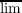#### Vol. 87, No. 2, 1980

 Recent Issues Vol. 325: 1 Vol. 324: 1  2 Vol. 323: 1  2 Vol. 322: 1  2 Vol. 321: 1  2 Vol. 320: 1  2 Vol. 319: 1  2 Vol. 318: 1  2Online Archive Volume: Issue:The Journal Subscriptions Editorial Board Officers Contacts Submission Guidelines Submission Form Policies for Authors ISSN: 1945-5844 (e-only) ISSN: 0030-8730 (print) Special Issues Author Index To Appear Other MSP Journals
Almost-periodic functions with unbounded integral

### Russell Allan Johnson

Vol. 87 (1980), No. 2, 347–362
##### Abstract

Let B be an almost-periodic (a.p.) function with mean value zero. Let G(t) = 0tB(s)ds. The well-known theorem of Bohr states that G(t) is uniformly bounded iff G(t) is a.p. This theorem may be reformulated in the following way. Let Ω be the hull of B, and let ,R) be the flow on Ω defined by translation. Since B is a.p., Ω is a compact abelian topological group. There is a continuous b : Ω R and an ω0 Ω such that b(ω0 t) = B(t). I.e., b “extends B to Ω”. Then Bohr’s theorem is equivalent to the following: G(t) is bounded iff there is a continuous r : Ω R such that r(ω t) r(ω) = 0tb(ω s)ds (ω Ω,t R).

In this paper, we consider the case when G(t) is unbounded. Two results are obtained. The first is a generalization of Bohr’s theorem: let μ be (normalized) Haar measure on Ω, and let gω(t) = 0tb(ω s)ds (ω Ω,t R); thenn→∞12{t [n,n]|gω(t) I} > 0 for some compact I R and some ω Ω iff there exists a μ-measurable r : Ω R such that r(ω t) r(ω) = 0tb(ω s)ds (ω Ω,t R). Here γ is Lebesgue measure on R. Thus, r exists if some gω(t) is not too badly unbounded. This theorem is stated for the class of “minimal” functions (see below), which includes the a.p. ones.

Primary: 42A75
Secondary: 28D99
##### Milestones